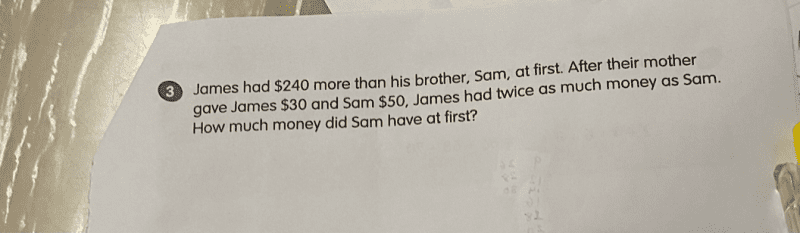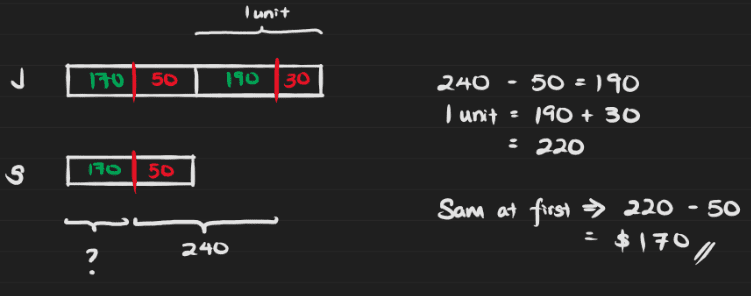# QuestionI would appreciate any help with this question. Thank you.Thank you very much.

0 Replies 0 Likes

I can only solve this by simultaneous equations.  I will be grateful if someone can supplement this by answering with models.

Let J be the amount of money James had at first.
Let S be the amount of money Sam had at first.

(1) J = S + 240

Now, J + 30 = 2 * (S + 50)
S0 J = 2S + 100 – 30
=> (2) J = 2S + 70

Placing (1) into (2),
S + 240 = 2S +70
S = 240 – 70 = 170

So Sam has \$170 at first #

Edit: Corrected calculation.  Adwin is correct.